# NEET 2020 Chemistry Paper With Solutions September 13

NEET Question paper 2020 for chemistry is provided here along with solutions. Chemistry section is asked for 45 questions, 180 marks of the total 180 questions and 720 marks respectively. Questions appear from different sections of chemistry – Organic, Physical and Inorganic chemistry. For effective preparation of NEET, NEET subject experts at BYJU’S solve the question paper with accurate solutions along with a brief explanation to justify why it is the correct answer and why others are not. Get access to this exclusive set of question and answers for chemistry, provided along with a downloadable PDF format of the same right below.

### NEET 2020 - Chemistry (Sep 13)

Question 1. Identify compound X in the following sequences of reactions: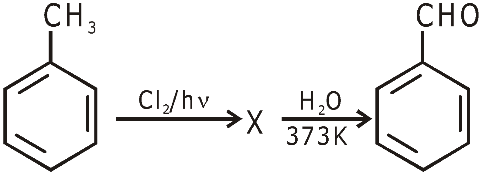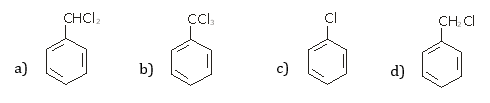Solution: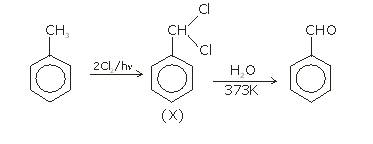Question 2. Identify a molecule which does not exist.

1. a)C2
2. b)O2
3. c)He2
4. d)Li2

Solution:

Diatomic molecule for which bond order is zero or negative does not exist.

 Molecule B.O. C2 2 O2 2 He2 Zero Li2 1

Question 3. Which of the following is a natural polymer?

3. c)cis-1,4-polyisoprene

Solution: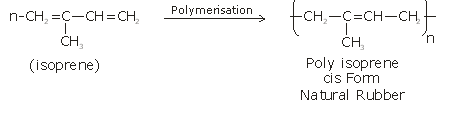Question 4. An increase in the concentration of the reactants of a reaction leads to change in:

1. a)threshold energy
2. b)collision frequency
3. c)activation energy
4. d)heat of reaction

Solution:

Collision frequency, as number of molecules per unit volume increases.

Question 5. Anisole on cleavage with HI gives: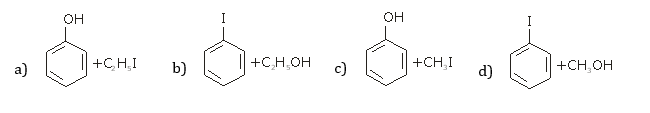Solution: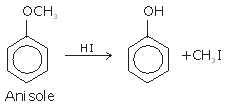Question 6. The number of protons, neutrons and electrons in, respectively, are:

1. a)71, 71 and 104
2. b)175, 104 and 71
3. c)71, 104 and 71
4. d)104, 71 and 71

Solution:

∴ no. of protons = 71

no. of neutrons = 175 – 71 = 104

no. of electrons = 71

Question 7. The calculated spin only magnetic moment of Cr2+ ion is:

1. a)5.92 BM
2. b)2.84 BM
3. c)3.87 BM
4. d)4.90 BM

Solution:

Cr2+→ 1s2 2s2 2p6 3s2 3p6 3d4

∴ n (number of unpaired electrons) = 4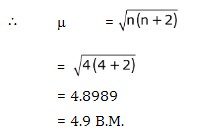Question 8. Match the following:

 Oxide Nature (a) CO (i) Basic (b) BaO (ii) Neutral (c) Al2O3 (iii) Acidic (d) Cl2O7 (iv) Amphoteric

Which of the following is correct option?

1. a)(iii) (iv) (i) (ii)
2. b)(iv) (iii) (ii) (i)
3. c)(i) (ii) (iii) (iv)
4. d)(ii) (i) (iv) (iii)

Solution:

 CO Neutral BaO Basic Al2O3 Amphoteric Cl2O7 Acidic

Question 9. Urea reacts with water to form A which will decompose to form B. B when passed through Cu2+ (aq), deep blue colour solution C is formed. What is the formula of C from the following?

1. a)Cu(OH)2
2. b)CuCO3.Cu(OH)2
3. c)CuSO4
4. d)[Cu(NH3)4]2+

Solution: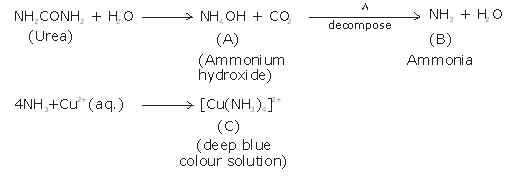Question 10. Match the following and identify the correct option.

 (a) CO(g) + H2(g) (i) Mg (HCO3)2 + Ca (HCO3)2 (b) Temporary hardness of water (ii) An electron deficient hydride (c) B2H6 (iii) Synthesis gas (d) H2O2 (iv) Non-planar structure

1. a)(iii) (iv) (ii) (i)
2. b)(i) (iii) (ii) (iv)
3. c)(iii) (i) (ii) (iv)
4. d)(iii) (ii) (i) (iv)

Solution:

⇒ CO(g) + H2(g) = Water gas or synthesis gas

⇒ Temporary hardness of water is due to bicarbonates of Ca2+& Mg2+

⇒ B2H6 is an electron deficient compound due to presence of banana bond.

⇒ H2O2 open book like structure which is non-planar

Question 11. The mixture which shows positive deviation from Raoult’s law is:

1. a)Acetone + Chloroform
2. b)Chloroethane + Bromoethane
3. c)Ethanol + Acetone
4. d)Benzene + Toluene

Solution:

Acetone + ethanol shows positive deviation from Raoult’s law. Pure ethanol possesses H-bonding and adding acetone to ethanol causes breaking of some H-bonds. This causes increase in observed vapour pressure.

Question 12. The freezing point depression constant (Kf) of benzene is 5.12 K kg mol–1. The freezing point depression for the solution of molality 0.078 m containing a non-electrolyte solute in benzene is (rounded off up to two decimal places):

1. a)0.40 K
2. b)0.60 K
3. c)0.20 K
4. d)0.80 K

Solution:

ΔTf = ikfm

⇒ ΔTf = 1 × 5.12 × 0.078

ΔTf = 0.3993

ΔTf = 0.40 K

Question 13. Which of the following set of molecules will have zero dipole moment?

1. a)Nitrogen trifluoride, beryllium difluoride, water, 1,3-dichlorobenzene
2. b)Boron trifluoride, beryllium difluoride, carbon dioxide, 1,4-dichlorobenzene
3. c)Ammonia, beryllium difluoride, water 1,4-dichlorobenzene
4. d)Boron trifluoride, hydrogen fluoride, carbon dioxide, 1,3-dichlorobenzene

Solution: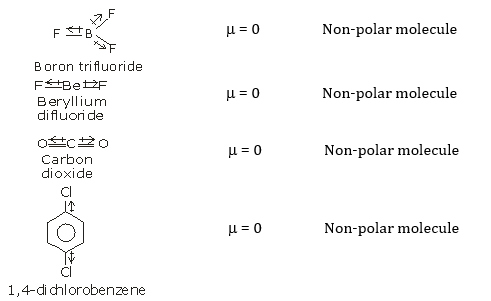Question 14. A tertiary butyl carbocation is more stable than a secondary butyl carbocation because of which of the following?

1. a)–R effect of –CH3 groups
2. b)Hyperconjugation
3. c)–I effect of –CH3 groups
4. d)+ R effect of –CH3 groups

Solution: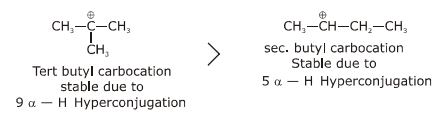Question 15. Find out the solubility of Ni (OH) 2 in 0.1 M NaOH. Given that the ionic product of Ni (OH)2 is 2×10–15.

1. a)1 × 10–13 M
2. b)1 × 108 M
3. c)2 × 10–13 M
4. d)2 × 10–8 M

Solution:

Ni(OH)2 Ni2+ + 2OH

– s 0.1 M

∴ Ksp = [Ni2+] [OH]2

⇒ ksp = s × (0.1)2

⇒ s =

⇒ s = 2 × 10–13 M

Question 16. Reaction between acetone and methyl magnesium chloride followed by hydrolysis will give:

1. a)Tert. butyl alcohol
2. b)Isobutyl alcohol
3. c)Isopropyl alcohol
4. d)Sec. butyl alcohol

Solution: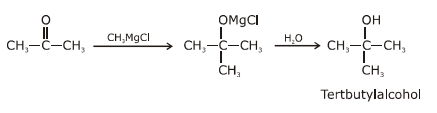Question 17. Which of the following amine will give the carbylamine test?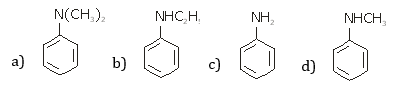Solution: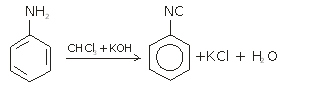Carbylamine reaction is gives by 1° amine & aniline.

Question 18. An alkene on ozonolysis gives methanal as one of the products. Its structure is: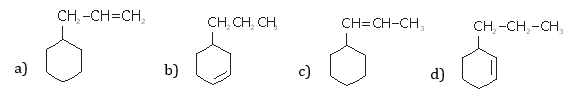Solution: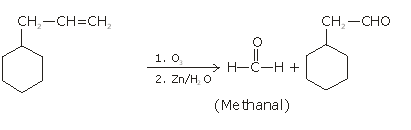Question 19. A mixture of N2 and Ar gases in a cylinder contains 7 g of N2 and 8 g of Ar. If the total pressure of the mixture of the gases in the cylinder is 27 bar, the partial pressure of N2 is:

[Use atomic masses (in g mol–1): N = 14, Ar = 40]

1. a)15 bar
2. b)18 bar
3. c)9 bar
4. d)12 bar

Solution:

According to Dalton’s law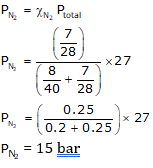Question 20. Which of the following is the correct order of increasing field strength of ligands to form coordination compounds?

1. a)F< SCN<C2O42-< CN
2. b)CN<C2O42-< SCN< F
3. c)SCN< F<C2O42-< CN
4. d)SCN< F< CN<C2O42-

Solution:

Fact from spectrochemical series.

Question 21. Paper chromatography is an example of:

1. a)Thin layer chromatography
2. b)Column chromatography
4. d)Partition chromatography

Solution:

It is an example of partition chromatography as mixture of substance is separated by partition.

Question 22. Sucrose on hydrolysis gives:

1. a)α-D-Glucose + β-D-Fructose
2. b)α-D-Fructose + β-D-Fructose
3. c)β-D-Glucose + α-D-Fructose
4. d)α-D-Glucose + β-D-Glucose

Solution: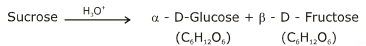Question 23. The rate constant for a first order reaction is 4.606 × 10–3 s–1. The time required to reduce 2.0 g of the reactant to 0.2 g is:

1. a)500 s
2. b)1000 s
3. c)100 s
4. d)200 s

Solution: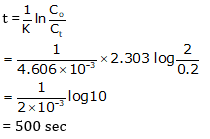Question 24. Reaction between benzaldehyde and acetophenone in presence of dilute NaOH is known as:

1. a)Cross Cannizzaro’s reaction
2. b)Cross Aldol condensation
3. c)Aldol condensation
4. d)Cannizzaro’s reaction

Solution: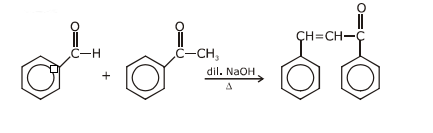Two different carbonyl compounds are treated with base known as cross-aldol condensation.

Question 25. Which of the following is not correct about carbon monoxide?

1. a)The carboxyhemoglobin (hemoglobin bound to CO) is less stable than oxyhemoglobin.
2. b)It is produced due to incomplete combustion.
3. c)It forms carboxyhemoglobin.
4. d)It reduces oxygen carrying ability of blood.

Solution:

CO when combine with hemoglobin it forms carboxyhemoglobin which more stable than oxyhemoglobin that is why it reduces oxygen carrying ability of blood.

Question 26. Hydrolysis of sucrose is given by the following reaction.

Sucrose + H2O Glucose + Fructose

If the equilibrium constant (Kc) is 2 × 1013 at 300 K, the value of ΔrG° at the same temperature will be:

1. a)8.314 J mol–1 K–1 × 300 K × ln (3 × 1013)
2. b)–8.314 J mol–1 K–1 × 300 K × ln (4 × 1013)
3. c)–8.314 J mol–1 K–1 × 300 K × ln (2 × 1013)
4. d)8.314 J mol–1 K–1 × 300 K × ln (2 × 1013)

Solution:

ΔG°= –RT ln K

⇒ΔG° = – 8.314 J mol–1 K–1 × 300 K × ln (2 × 1013)

Question 27. HCl was passed through a solution of CaCl2, MgCl2 and NaCl. Which of the following compound(s) crystallize (s)?

1. a)only MgCl2
2. b)NaCl, MgCl2 and CaCl2
3. c)Both MgCl2 and CaCl2
4. d)Only NaCl

Solution:

MgCl2 has least solubility among CaCl2, MgCl2 & NaCl that is why it crystallizes first on passing HCl.

irst on passing HCl.

Question 28. An element has a body centered cubic (bcc) structure with a cell edge of 288 pm. The atomic radius is: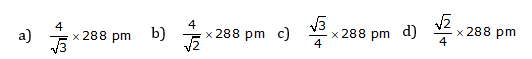Solution: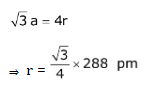Question 29. Which of the following oxoacid of sulphur has –O–O– linkage?

1. a)H2S2O8, peroxodisulphuric acid
2. b)H2S2O7, pyrosulphuric acid
3. c)H2SO3, sulphurous acid
4. d)H2SO4, sulphuric acid

Solution: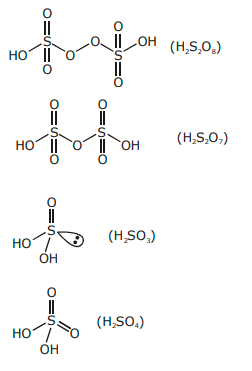Question 30. Identify the incorrect statement.

1. a)Interstitial compounds are those that are formed when small atoms like H, C or N are trapped inside the crystal lattices of metals.
2. b)The oxidation states of chromium in CrO42- and are not the same.
3. c)Cr2+(d4) is a stronger reducing agent than Fe2+(d6) in water.
4. d)The transition metals and their compounds are known for their catalytic activity due to their ability to adopt multiple oxidation states and to form complexes.

Solution:

1. Interstitial compounds are those compounds that are formed when small atoms like H, B, C or N are trapped inside crystal lattices of metals.

(2) Oxidation state of chromium in CrO42–& Cr2O72–are +6 (same)

(3) Fact

Cr2+ is stronger reducing agent than Fe2+ in water on the basis of standard reduction potential.

(4) Transition metals are one of the best available catalyst due to their ability to adopt multiple oxidation states & to form complexes.

Question 31. Which of the following is a cationic detergent?

1. a)Cetyltrimethyl ammonium bromide
2. b)Sodium dodecylbenzenesulphonate
3. c)Sodium lauryl sulphate
4. d)Sodium stearate

Solution:

Cetyltrimethyl ammonium bromide is a cationic detergent as it’s hydrophillic part is a quaternary ammonium ion.

1. a)q < 0, ΔT = 0 and w = 0
2. b)q > 0, ΔT > 0 and w > 0
3. c)q = 0, ΔT = 0 and w = 0
4. d)q = 0, ΔT < 0 and w > 0

Solution:

For an adiabatic process, q = 0

∴ w = 0

& from First law of Thermodynamics, ΔE = 0

Or ΔT = 0

Question 33. On electrolysis of dilute sulphuric acid using platinum (Pt) electrode, the product obtained at anode will be:

1. a)H2S gas
2. b)SO2 gas
3. c)Hydrogen gas
4. d)Oxygen gas

Solution:

Anode: 2H2O ⎯→ O2 + 4H+ + 4e

Cathode: 2H+ + 2e⎯→ H2(g)

∴oxygen gas will be obtained at anode.

Question 34. Identify the correct statement from the following:

1. a)Vapour phase refining is carried out for Nickel by Van Arkel method.
2. b)Pig iron can be moulded into a variety of shapes.
3. c)Wrought iron is impure iron with 4% carbon.
4. d)Blister copper has blistered appearance due to evolution of CO2.

Solution:

(1) Vapour phase refining is carried out for nickel by Mond process.

(2) Pig iron is molten iron obtained from blast furnace after smelting it can be moulded into variety of shapes.

(3) Wrought iron is the purest form of iron which has less than 4% carbon.

(4) Blister copper has blistered appearance due to evolution of SO2.

Question 35. Which of the following is a basic amino acid?

1. a)Tyrosine
2. b)Lysine
3. c)Serine
4. d)Alanine

Solution: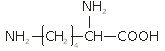(Lysine)

Basic part is more than acidic part so lysine is basic amino acid.

Question 36. Identify the incorrect match.

 Name IUPAC Official Name (a) Unnilunium (i) Mendelvium (b) Unniltrium (ii) Lawrencium (c) Unnilhexium (iii) Seaborgium (d) Unununnium (iv) Darmstadtium

1. a)(c), (iii)
2. b)(d), (iv)
3. c)(a), (i)
4. d)(b), (ii)

Solution:

101 - Unnilunium - Mendelevium

103 - Unniltrium - Lawrencium

106 - Unnilhexium - Seaborgium

111 - Unununium - Roentgenium

Question 37. Which of the following alkane cannot be made in good yield by Wurtz reaction?

1. a)n-Heptane
2. b)n-Butane
3. c)n-Hexane
4. d)2,3-Dimethylbutane

Solution:

Wurtz reaction is used to prepare symmetrical alkane.

Question 38. Elimination reaction of 2-Bromo-pentane to form pent-2-ene is:

(a) β-Elimination reaction

(b) Follows Zaitsev rule

(c) Dehydrohalogenation reaction

(d) Dehydration reaction

1. a)(b), (c), (d)
2. b)(a), (b), (d)
3. c)(a), (b), (c)
4. d)(a), (c), (d)

Solution: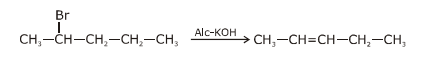In the reaction

(i) Dehydrohalogenation

(ii) β - elimination reaction

(iii) Follows zaitsev rule (highly substituted alkene is formed as major product)

Question 39. The number of Faradays (F) required to produce 20g of calcium from molten CaCl2 (Atomic mass of Ca = 40 g mol–1) is:

1. a)3
2. b)4
3. c)1
4. d)2

Solution:

EquivalentCa = Equivalentcurrent

⇒ No. of Faradays = 1

Question 40. Which one of the followings has maximum number of atoms?

1. a)1g of O2(g) (Atomic mass of O=16)
2. b)1g of Li(s) (Atomic mass of Li=7)
3. c)1g of Ag(s) (Atomic mass of Ag = 108)
4. d)1g of Mg(s) (Atomic mass of Mg = 24)

Solution:

(1) no. of atoms =

(2) no. of atoms =

(3) no. of atoms =

(4) no. of atoms =

∴ 1 gm of Li is having maximum no. of atoms.

Question 41. For the reaction, 2Cl(g) → Cl2(g), the correct option is:

1. a)ΔrH< 0 and ΔrS> 0
2. b)ΔrH< 0 and ΔrS< 0
3. c)ΔrH> 0 and ΔrS> 0
4. d)ΔrH> 0 and ΔrS< 0

Solution:

2Cl(g) ⎯→ Cl2(g) + Heat

Due to bond formation stability increases which results in release of heat.

∴ ΔHr = –ve or exothermic process

ΔHr< O

& ΔS < O, because number of Cl atoms decreases in the formation of Cl2(g)

Question 42. Identify the correct statements from the following:

(a) CO2(g) is used as refrigerant for ice-cream and frozen food.

(b) The structure of C60 contains twelve six carbon rings and twenty-five carbon rings.

(c) ZSM-5, a type of zeolite, is used to convert alcohols into gasoline.

(d) CO is colorless and odourless gas.

1. a)(b) and (c) only
2. b)(c) and (d) only
3. c)(a), (b) and (c) only
4. d)(a) and (c) only

Solution:

⇒ CO2 (s) is not used as refrigerant for ice-cream & frozen food.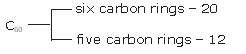⇒ ZSM-5 zeolite used to convert alcohols into gasoline

⇒ CO is colorless & odorless gas

Question 43. Measuring Zeta potential is useful in determining which property of colloidal solution?

1. a)Stability of the colloidal particles
2. b)Size of the colloidal particles
3. c)Viscosity
4. d)Solubility

Solution:

A large +ve or –ve value of Zeta potential indicates good stability of the colloidal particles.

Question 44. What is the change in oxidation number of carbons in the following reaction?

CH4(g) + 4Cl2(g) → CCl4(l) + 4HCl(g)

1. a)–4 to +4
2. b)0 to –4
3. c)+4 to +4
4. d)0 to +4

Solution: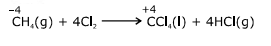Question 45. The following metal ion activates many enzymes, participates in the oxidation of glucose to produce ATP and with Na, is responsible for the transmission of nerve signals.

1. a)Calcium
2. b)Potassium
3. c)Iron
4. d)Copper

Solution:

Solution: Potassium

### NEET 2020 Chemistry Paper With Solutions September 13